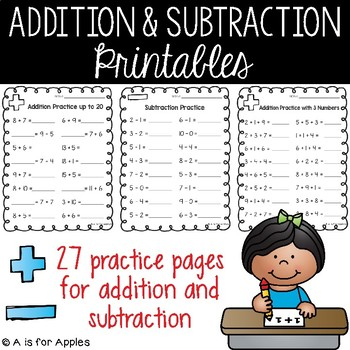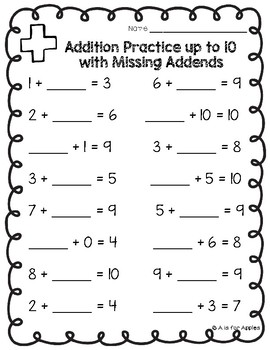# Addition & Subtraction Practice PagesK - 1st, Homeschool
Subjects
Standards
Resource Type
Formats Included
• PDF
Pages
30 pages

### Description

28 pages of differentiated addition and subtraction practice. Just print and go! These pages are great for morning work, independent math practice, homework, and more. Use these to help students with their addition and subtraction fluency in Kindergarten and 1st grade.

There are 14 addition pages and 14 subtraction pages included.

-practice to 10

-practice to 20

-doubles

-doubles +1

-facts of 10

-commutative property

Subtraction topics include:

-difference unknown from 10

-different unknown from 20

-finding 1 less

-finding 2 less

-facts of 10

-dice subtraction

-domino subtraction

Total Pages
30 pages
N/A
Teaching Duration
N/A
Report this Resource to TpT
Reported resources will be reviewed by our team. Report this resource to let us know if this resource violates TpT’s content guidelines.

### Standards

to see state-specific standards (only available in the US).
Determine the unknown whole number in an addition or subtraction equation relating three whole numbers. For example, determine the unknown number that makes the equation true in each of the equations 8 + ? = 11, 5 = ▯ - 3, 6 + 6 = ▯.
Add and subtract within 20, demonstrating fluency for addition and subtraction within 10. Use strategies such as counting on; making ten (e.g., 8 + 6 = 8 + 2 + 4 = 10 + 4 = 14); decomposing a number leading to a ten (e.g., 13 - 4 = 13 - 3 - 1 = 10 - 1 = 9); using the relationship between addition and subtraction (e.g., knowing that 8 + 4 = 12, one knows 12 - 8 = 4); and creating equivalent but easier or known sums (e.g., adding 6 + 7 by creating the known equivalent 6 + 6 + 1 = 12 + 1 = 13).
Understand subtraction as an unknown-addend problem. For example, subtract 10 – 8 by finding the number that makes 10 when added to 8.
Apply properties of operations as strategies to add and subtract. If 8 + 3 = 11 is known, then 3 + 8 = 11 is also known. (Commutative property of addition.) To add 2 + 6 + 4, the second two numbers can be added to make a ten, so 2 + 6 + 4 = 2 + 10 = 12. (Associative property of addition.)
Fluently add and subtract within 5.